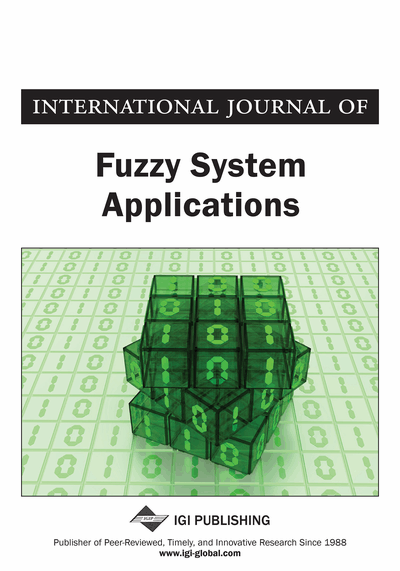# Determining of Level Sets for a Fuzzy Surface Using Gröbner Basis

Hamed Farahani (Chabahar Maritime University, Sistan Va Baluchestan Iran, Iran), Sajjad Rahmany (School of Mathematics and Computer Sciences Damghan University, Damghan, Iran), and Abdolali Basiri (School of Mathematics and Computer Sciences Damghan University, Damghan, Iran)
DOI: 10.4018/IJFSA.2015040101
Available
\$29.50
No Current Special Offers

## Abstract

In this paper, a manner to determine the level sets of a fuzzy surface using the benefits of Gröbner basis is presented. Fuzzy surfaces are constructed from incomplete datasets or from data that contain uncertainty which has not statistical nature. The authors firstly define the concept of level sets for the fuzzy surfaces. Then, employing Gröbner bases benefits a criterion is proposed for when the level sets of the fuzzy surface are nonempty sets. Moreover, a new algorithm is designed to determine the level sets. The big advantage of the proposed method lies in the fact that it attains all members of the level sets of the fuzzy surface at a time. Finally, some applied numerical examples are illustrated to demonstrate the proficiency of the given approach.
Article Preview
Top

## 1. Introduction

Fuzzy surfaces play a major role in various areas of sciences such as geosciences (Burrough and McDonnell 1998, Caha et al. 2012, Janoska and Dvorsky 2012, Lodwick 2008, Waelder 2007), medical sciences (Dubey and Nagpal 2011). In these areas, the information used for modelling is often incomplete. For example points used to the model surface are only samples from the set of all points on the surface and their precision can be unknown. From such inputs it is not possible to create precise and absolutely certain surface. It is necessary to include the uncertainty, that has origin in both input data and interpolation process, in the surface. Since none of the mentioned types of uncertainty is of statistical nature it is convenient to conceptualize the uncertainty by an alternative method i.e. fuzzy set theory. Fuzzy set theory and fuzzy logic dispose of methods and tools for modelling surfaces based on biased input data. Especially fuzzy numbers are suited for modelling surfaces with uncertainty. Triangular and trapezoidal fuzzy numbers are the most common ones that are used in applications because of their easy implementation.

In this work, a novel approach is presented dealing with fuzzy surfaces of arbitrary degree. Firstly, consider an arbitrary fuzzy fixed constant is considered. Then, with equalization the fuzzy fixed constant and the fuzzy surface (withreal independent variables) a multivariate fuzzy polynomial equation will be obtained. The set of all ordered-tuples inthat satisfy this multivariate fuzzy polynomial equation is called the level set for the fuzzy surface. Our aim in this work is to determine the level sets for the fuzzy surfaces using Gröbner basis. The presented idea in this paper is to compute the-cuts of the multivariate fuzzy polynomial equation and obtain its parametric form with respect toand the sign of variables. Then, the parametric form of the fuzzy polynomial equation with respect tois collected and polynomials asare obtained, where. We compute a Gröbner basisfor the ideal generated bywith respect to the lexicographical order. The basis has an upper triangular structure. Then the system can be solved using the forward substitution. We will show that the set of solutions of the Gröbner basis system and the level set of the fuzzy surface are equal. Also, a criterion is proposed based on Gröbner basis for when the level sets of the fuzzy surfaces are empty sets.

The structure of this paper is organized as follows. Section 2 is divided to two subsections. Some required definitions and results about fuzzy numbers is given in the first subsection. The required concepts and results about the Gröbner basis are presented in the second subsection. Section 3 is divided to two subsections. Some required definitions and results about fuzzy surfaces is given in the first subsection. In the second subsection the main idea for determining the level sets for the fuzzy surfaced is presented. Furthermore, an algorithm is proposed to determine the level sets. Some examples are given to illustrate the algorithm in Section 4. Finally, conclusions are presented in Section 5.

## Complete Article List

Search this Journal:
Reset
Volume 12: 1 Issue (2023)
Volume 11: 4 Issues (2022)
Volume 10: 4 Issues (2021)
Volume 9: 4 Issues (2020)
Volume 8: 4 Issues (2019)
Volume 7: 4 Issues (2018)
Volume 6: 4 Issues (2017)
Volume 5: 4 Issues (2016)
Volume 4: 4 Issues (2015)
Volume 3: 4 Issues (2013)
Volume 2: 4 Issues (2012)
Volume 1: 4 Issues (2011)
View Complete Journal Contents Listing